Trial ends in

# Determining the Mass Percent Composition in an Aqueous Solution

### Overview

Source: Laboratory of Dr. Neal Abrams — SUNY College of Environmental Science and Forestry

Determining the composition of a solution is an important analytical and forensic technique. When solutions are made with water, they are referred to as being aqueous, or containing water. The primary component of a solution is referred to as the solvent, and the dissolved minor component is called the solute. The solute is dissolved in the solvent to make a solution. Water is the most common solvent in everyday life, as well as nearly all biological systems. In chemistry labs, the solvent may be another liquid, like acetone, ether, or an alcohol. The solute can be a liquid or a solid, but this experiment only addressesthe determination of solids.

### Principles

When a solute is dissolved in a solvent, the mass of the resultant solution is a sum of the mass of the solute and the solvent. This follows the Law of Conservation of Mass:This differs from the addition of volumes, which is notadditive, since molecules of the solute fit into open spaces between the molecules of the solvent and keeps the total volume lower than expected. Determining percent by mass is a simple and important technique for analyzing a solution's makeup. By definition, a percent is a fractional,unitless value of one component compared to the whole. In this case, the percent by mass can be represented mathematically as: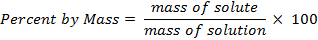Although percent by mass is unit-less, this value is sometimes represented with the units (w/w) to convey that the comparison is by mass (weight) only and not by volume. Percent by volumes are indicated as weight per volume (w/v) or volume per volume (v/v). In w/w calculations, it is important to note that the denominator is the mass of the entire solution, not the solvent. While percent by mass is used to determine solution concentration, percent by mole is typically used to calculate the percent of anelement or group in a molecule. It is difficult to calculate percent by mole in a solution, since the total moles of solute and solvent would need to be known. If those values were known, the overall calculation would be irrelevant.

In some cases, obtaining the mass of the solvent or solute individually can be difficult or impractical. In these cases, the density of a solution can be used to determine mass percent by first producing a calibration curve of solutions with known compositions. This first requires the following to be known: the identity of the solute anda reasonable range of the solution's concentration.More complex analytical methods are required when more than one solute is present in solution. For example, selective precipitation or ion exchange could be used to analyze for a solute of interest. For single solute solutions, enough material must be available to create a series of solutions of known concentration.Two percent by mass methods are demonstrated here.

### Procedure

1. Percent by Mass - Direct

1. Place a small volume of a solution into a clean and oven-dried beaker or crystallization dish.
2. After accurately determining the precise total mass of the solution, heat the beaker or dish on a hotplate or in an oven to drive off the water. Slow evaporation is the best method, as boiling can result in splattering of the solution.
3. Once the solvent has evaporated, cool the remaining solid (solute) and determine the mass.
4. Calculate the mass percent as: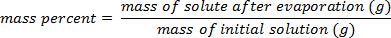2. Percent by Mass - Using a Calibration Curve

1. Make a series of standard solutions by dissolving known quantities of solute into a solvent. Five standards are recommended and should range from the minimum to the maximum expected percent compositions.
1. If the approximate value is unknown, produce a series of solutions ranging from 0% through the solubility maximum of the solute in water. Reference tables are available to indicate the maximum solubility for many solids in water over a variety of temperature ranges.
2. For example, to produce a 35% (w/w) sodium chloride (NaCl) solution, add 35 g to a flask, then add 65 g (or 65 mL, as volume can also be used when measuring pure water since the density is known to be 1.0 g/mL at ambient temperatures) of water, and mix the solution until the solid is dissolved. Because mass is additive, this would be the same as adding enough water so the total solution has a mass of 100 g.
2. After measuring the mass of a precise volume of each of the standard solutions, calculate the density as:3. Data Analysis

1. Plot the density values against the percentage by mass of the standard solutions and determine the slope of the line. This slope corresponds to the density vs. mass percent of the solution, fitting a linear equation, y = mx + b (Figure 1).
2. Calculate the density of the unknown sample solution by obtaining the mass of the specific volume of solutions. Now that the slope of the line is known, determine the percent by mass of solute by solving for "x" using the measured density and slope from the calibration curve.

Determining and understanding the composition of a solution is fundamental in a wide range of chemistry techniques. A solution is a homogeneous mixture, composed of a substance dissolved in a liquid. The dissolved substance is called the solute, and the bulk fluid is called the solvent. Solutions can be described in several ways — first, by the type of solvent used to dissolve the solute. Aqueous solutions utilize water as the solvent. Organic solvents, such as chloroform, acetonitrile, or acetone, are used to make organic solutions, depending on the properties of the solute.

Solutions are also described by the amount of solute dissolved in a certain volume of the solvent, or the concentration. For more information, see this collection's video on "Solutions and Concentrations".

One way solution concentration can be calculated is based on the mass of each component of the solution, called mass percent. This video will introduce the mass percent of solutions, and demonstrate how to determine the value in the laboratory.

The amount of solute in the solvent can be expressed in a number of ways. First, mass percent is calculated as the mass of the solute divided by the total mass of the solution. Mass percent is written as percent weight/weight, as it is sometimes referred to as weight percent. However, the term mass percent is generally regarded as correct.

The amount of solute in a solution can also be expressed in terms of mass percent by volume, commonly used in biology, and is calculated as the total mass of the solute divided by the volume of the solution. This unit is not a true percent, as it is not a dimensionless quantity. Similarly, volume percent is also used, where the volume of the solute is divided by the total volume of the solution.

Mass percent differs from volume percent, as only mass is an additive quantity. The mass of the solution is the sum of the components. Volume, on the other hand, isn't always additive, as molecules of the solute will fit into open spaces between molecules of solvent, resulting in less volume than expected.

Several simple methods can be used to determine the mass percent of a solution in the laboratory. First, the direct method requires recording the total mass of solution, and then evaporating off the solvent. The solid left behind is the solute, which is then weighed and used to calculate mass percent.

Density can also be used to determine the mass percent of an unknown concentration. First, a series of standard solutions are prepared, with a range of solute amounts. Select standards that range in concentration from no solute, to the maximum solubility of the solute. The density is then calculated for each standard, and compared to that of the sample. Now that the basics of mass percent calculations have been outlined, lets take a look at calculating mass percent using the direct method and the density method in the laboratory.

First, weigh a clean and oven-dried beaker or crystallization dish, and record the mass.

Place a small volume of the solution into the dish, and accurately weigh the solution and dish. Subtract the weight of the dish, and record the mass of the solution.

Next, place the dish on a hotplate or in an oven to drive off the water. Slow evaporation is ideal, as boiling can result in splattering of the solution, and loss of solute. Once the solvent has evaporated, cool the remaining solid, which is the solute. Accurately determine the mass. Calculate the mass percent by dividing the mass of the solute after evaporation by the mass of the initial solution.

First, prepare five standards, with percent compositions ranging from the minimum to the maximum of the expected unknown percent composition. Do not exceed the solubility of the system. Calculate the amount of solute and solvent needed to prepare the percent compositions.

Weigh the solute, and then dissolve it in the specific weight of solvent.

Next, measure the mass of a precise volume of each standard solution. Then calculate density for each solution. Do the same for the unknown solution. See this collection's video on density for more information.

Plot the density values of the standard solutions against the mass percentages. In this example, a set of sodium chloride standards was prepared with mass percent compositions ranging from 5 – 25% of solute in solution. After plotting the data, apply a linear trend-line, and determine the equation, where y is density and x is mass percent composition. Use the density of the unknown solution, in this case 1.053 g/mL, to determine its mass percent. Solving for x, it was found to be 7.4%.

Now that you've seen the basics of determining the mass percent of aqueous solutions, lets take a look at some instances where the concentration of a solution must be known.

Buffer solutions are commonly used in many laboratory experiments, especially in biological applications. Buffer solutions contain a specific concentration of dissolved weak acids or base, and their conjugates, enabling it to maintain and resist changes in pH. Often, buffers are prepared using a mixture of many salts, including those with phosphate groups that maintain a constant pH in the range of 7.2 – 7.6.

Mass percent is also used in order to separate complex mixtures, as with this example of a density gradient. For this, solutions with a range of mass percent are prepared, and then layered sequentially from high mass percent to low mass percent. This creates a density gradient in the tube. The sample is then loaded onto the top of the gradient, and centrifuged. The components of the mixture are then separated based on how far they travel down the tube.

Understanding mass percent is not only critical to chemistry applications, but also to environmental studies. Soil moisture content is important to understand in order to identify environmental conditions that may influence ecosystems. Soil moisture content is a mass percent that describes the amount of water held within the soil. Moisture content is easily found by weighing the moist soil, desiccating the soil it in an oven, and reweighing.

You've just watched JoVE's introduction to determining the mass percent of an aqueous solution. You should now understand the importance of mass percent, its use in the laboratory, and how to determine it for an aqueous solution.

Thanks for watching!

### Results

Using the example shown in Figure 1, a set of sodium chloride standards was prepared with mass percent compositions of 5.000%, 10.00%, 15.00%, 20.00%, and 25.00% of solute in solution. The measured densities were 1.025, 1.042, 1.060, 1.070, and 1.090 g/mL, respectively. After plotting these data, a linear trendline is applied, fitting the equation y = 3.446 x 10-3x + 1.0048, where y is the density and x is the mass percent composition.

Next, the volume of 10.00 g of solution with unknown percent composition is measured and determined to be 9.497 mL. By dividing the mass by the volume, the density is then calculated as 1.053 g/mL. Inserting the density value into the linear equation, the mass percent is determined as x: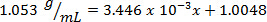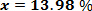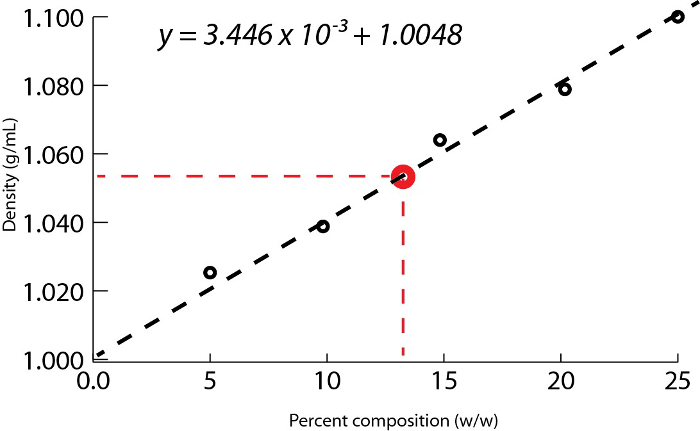Figure 1. Aqueous sodium chloride solution density as a function of percent composition by mass.

### Applications and Summary

The percentage of sugar in soda, could easily be determinedusing the principle of mass percent composition. The procedure for doing this experiment would be to measure the mass and volume of degassed soda (no bubbles) and to calculate the solution's density. A calibration curve of density vs. percent by mass for several standard sucrose (sugar) solutionswould need to be created, and then that calibration could be used to solve for the percent of sucrose in the soda. One assumption is that sucrose is the major contributor to a change in density for soda, which is true for non-sugar-free soda.

Seawater is composed of several different salts dissolved in water; for example, NaCl, MgCl2, and NaBr2. While it would be difficult to determine mass percent using a calibration curve, evaporation provides a simple solution to the problem. By taking a known mass of seawater and evaporating it, the mass can be determined by the amount of solute remaining after the solvent is evaporated. Then, the mass of solute needs to be divided by the total mass of the original solution to calculate the mass percent of salts (dissolved solids) in seawater.

There are a variety of ionic compounds that have water molecules and are referred to as hydrates. The number of water molecules on a compound can be determined by percent composition. For example, cobalt (II) chloride is normally blue, but turns pink when it is hydrated. If 5.0 grams of pink cobalt (II) chloride hydrate is heated to the point it turns blue, then the final mass of the blue solid is recorded as 2.73. The difference, 2.27 g, corresponds to the mass of water that was on the 5 g of hydrated solid. The original sample was 45.4 % water by mass. By converting mass values to moles, it can be calculated that 0.02101 moles of cobalt chloride (129.93 g/mol) and 0.126 moles of water (18 g/mol) are present. Dividing by the smallest value, it can be determined that 6 moles of water are present for every one mole of cobalt chloride.X